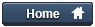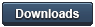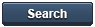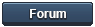### Gammon Forum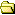Entire forum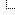Electronics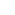Microprocessors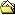Using a 74HC595 output shift register as a port-expander

Using a 74HC595 output shift register as a port-expander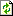Refresh page

Posted by Nick Gammon   Australia  (22,921 posts)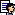bio   Forum Administrator
Date Tue 31 Jan 2012 08:33 PM (UTC)

Amended on Fri 10 Oct 2014 12:06 AM (UTC) by Nick Gammon

Message
 A cheap and simple way of expanding your processor's output capability is by using a "shift register" like the 74HC595 described here. Tip: A complementary device is the 74HC165 input shift register mentioned in the post below: http://www.gammon.com.au/forum/?id=11979 Example of it in use, displaying 8 LEDs: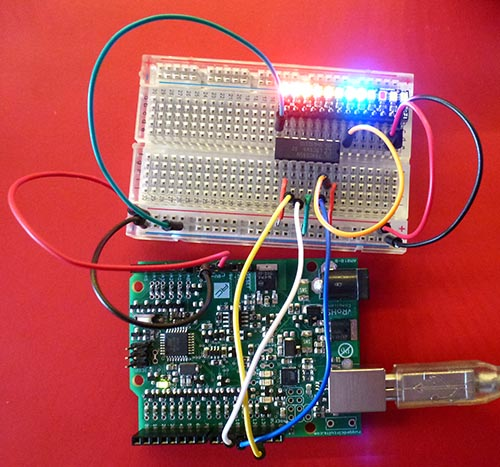Pin outs The pin-outs for the 74HC595 are: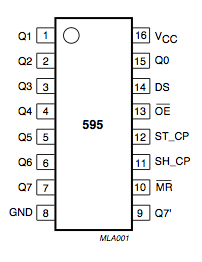You can "daisy-chain" them to connect multiple ones together, thus giving you 8, 16, 24, 32 or more extra output ports, by simply connecting the "overflow" bit of one register to the "data in" of the next. Schematic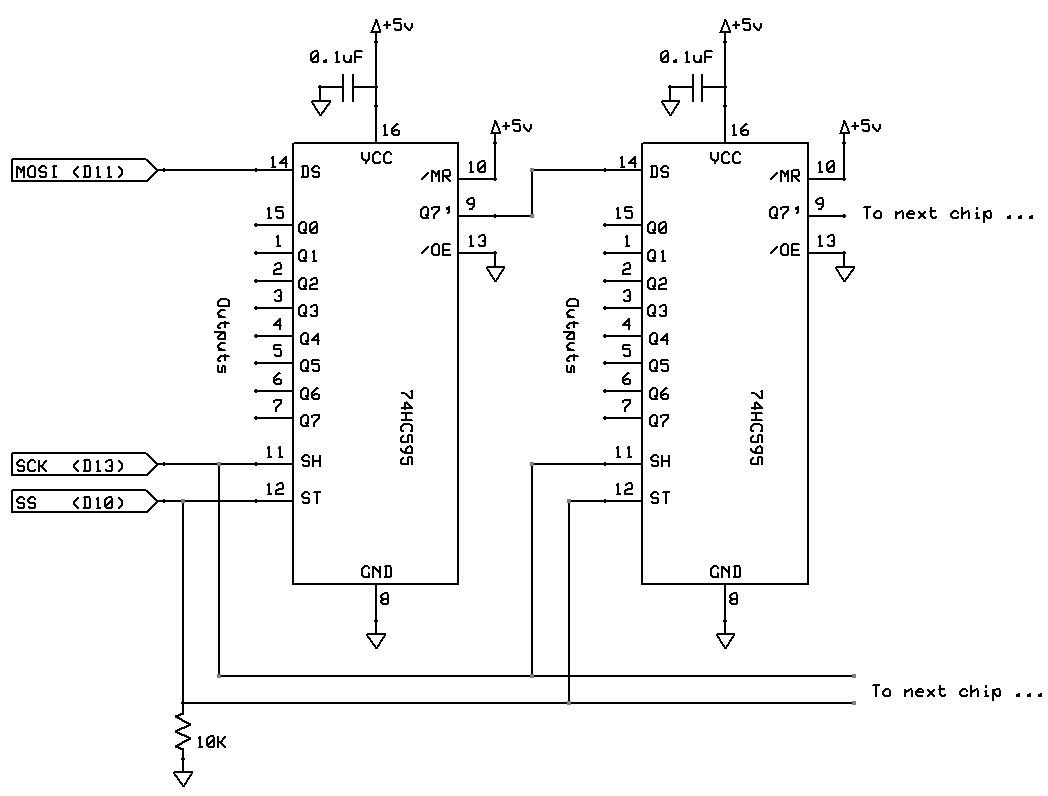The 10K pull-down resistor on the SS (Slave Select) is designed to keep the registers from clocking in bits while the main processor is booting, and the SS line might be "floating" and in an indeterminate state. Master reset I have tied /MR (master reset) high, so the chips are never in a reset state. If you needed to reset them from time to time you could parallel up those pins and connect them to a processor pin. Output enable I have tied /OE (output enable) low, so the chips are always in output mode. If you needed to have them high impedance (neither high nor low) you could parallel up those pins and connect them to a processor pin (eg, D9). Program code It's extremely simple to send data to the shift register. You can use SPI to do the hard work: `````` #include const byte LATCH = 10; void setup () { SPI.begin (); } // end of setup byte c; void loop () { c++; digitalWrite (LATCH, LOW); SPI.transfer (c); digitalWrite (LATCH, HIGH); delay (20); } // end of loop `````` More details about SPI here: http://gammon.com.au/spi The call to SPI.transfer() sends a byte to the register (a bit at a time) and then bringing the LATCH (otherwise known as SS or ST) line high causes the data to be transferred from the register's internal memory to the output pins. Pin connections Pin connections for Uno and similar: `````` // Chip pin 14 (DS) goes to MOSI (D11) // Chip pin 11 (SH) goes to SCK (D13) // Chip pin 12 (ST) goes to SS (D10) (or any other pin by changing LATCH) `````` Pin connections for Mega2560: `````` // Chip pin 14 (DS) goes to MOSI (D51) // Chip pin 11 (SH) goes to SCK (D52) // Chip pin 12 (ST) goes to SS (D10) (or any other pin by changing LATCH) `````` Daisy chaining To send more than 8 bits you simply transfer more than once before latching. So if you had four devices chained together you might do this: `````` digitalWrite (LATCH, LOW); SPI.transfer (0xAB); SPI.transfer (0xCD); SPI.transfer (0xEF); SPI.transfer (0x42); digitalWrite (LATCH, HIGH); `````` Once more than 8 bits arrive at the first register they "overflow" out the Q7' port and go into the DS (data in) port of the next device. And so on, if there are more than 16 bits. So what eventually happens is that the 0xAB byte ends up in the device the furthest down the chain. Effectively: `````` Sent data Data in devices (nothing) xx xx xx xx AB AB xx xx xx CD CD AB xx xx EF EF CD AB xx 42 42 EF CD AB (latch) Commit to output pins on all devices `````` Where "xx" represents whatever was there before. Thus the furthest device down the chain gets whatever byte was sent first. SPI is very fast, it takes around 3 uS to send one byte, so sending all 4 bytes would only take around 12 uS. Bit-banged SPI code The code below uses "bit banged" SPI rather than the hardware SPI. This lets you use any pins for the shift register, perhaps keeping the SPI pins free for an SD card or other device. `````` #include bitBangedSPI bbSPI (8, bitBangedSPI::NO_PIN, 9); // MOSI, MISO, SCK const byte LATCH = 10; void setup () { bbSPI.begin (); pinMode (LATCH, OUTPUT); } // end of setup byte c; void loop () { c++; digitalWrite (LATCH, LOW); bbSPI.transfer (c); digitalWrite (LATCH, HIGH); delay (20); } // end of loop `````` You need to install the "bitBangedSPI" library (link below) into your libraries folder: http://www.gammon.com.au/Arduino/bitBangedSPI.zip Note, the LEDs shown have built-in current limiting resistors. You would not normally put LEDs directly onto the output of the 595 chip, as the current drain would be too high. - Nick Gammon www.gammon.com.au, www.mushclient.com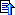top

Posted by Nick Gammon   Australia  (22,921 posts)bio   Forum Administrator
Date Reply #1 on Wed 02 May 2012 06:11 AM (UTC)

Amended on Tue 26 Nov 2013 01:16 AM (UTC) by Nick Gammon

Message
 Example of 4 shift register chips breadboarded with 32 LEDs: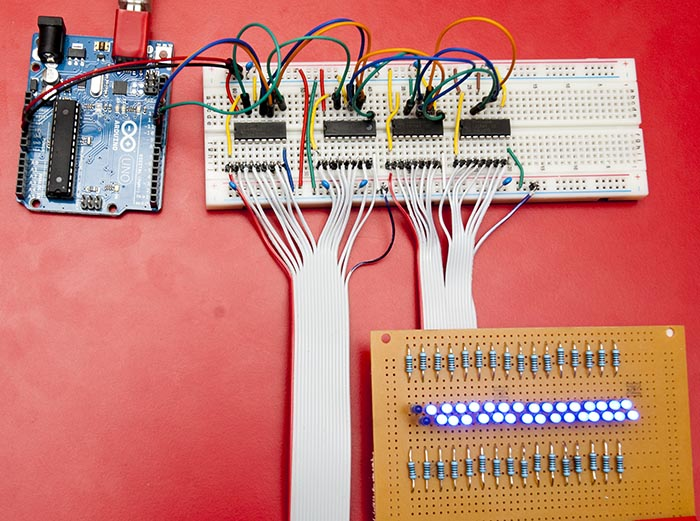There are about 8 x 0.1 uF decoupling capacitors there between +5V and Gnd, to keep it all stable. There are 1K resistors on the board in series with the LEDs. Video of this in action: Sketch to make the LEDs light up in sequence: `````` // Demo sketch to turn sequence up and down bits in 4 shift registers // Author: Nick Gammon // Date: 2 May 2012 #include const byte LATCH = 10; const byte numberOfChips = 4; byte LEDdata [numberOfChips]; // initial pattern void refreshLEDs () { digitalWrite (LATCH, LOW); for (byte i = 0; i < numberOfChips; i++) SPI.transfer (LEDdata [i]); digitalWrite (LATCH, HIGH); } // end of refreshLEDs void setup () { SPI.begin (); } // end of setup void showPattern (const unsigned int p1, const unsigned int p2) { LEDdata  = highByte (p1); LEDdata  = lowByte (p1); LEDdata  = highByte (p2); LEDdata  = lowByte (p2); refreshLEDs (); delay (30); } // end of showPattern void loop () { unsigned int pattern; pattern = 1; for (int i = 0; i < 16; i++) { showPattern (pattern, pattern); pattern <<= 1; } pattern = 0x8000; for (int i = 0; i < 16; i++) { showPattern (pattern, pattern); pattern >>= 1; } pattern = 1; for (int i = 0; i < 16; i++) { showPattern (~pattern, ~pattern); pattern <<= 1; } pattern = 0x8000; for (int i = 0; i < 16; i++) { showPattern (~pattern, ~pattern); pattern >>= 1; } } // end of loop `````` Another sketch that lets you choose from the serial monitor which LED to turn on. [EDIT] However see further down for a more sophisticated version. `````` // Demo sketch to turn on or off bits in a set of shift registers // Author: Nick Gammon // Date: 2 May 2012 #include const byte LATCH = 10; const byte numberOfChips = 4; const byte maxLEDs = numberOfChips * 8; byte LEDdata [numberOfChips] = { 0 }; // initial pattern void refreshLEDs () { digitalWrite (LATCH, LOW); for (int i = numberOfChips - 1; i >= 0; i--) SPI.transfer (LEDdata [i]); digitalWrite (LATCH, HIGH); } // end of refreshLEDs // how much serial data we expect before a newline const unsigned int MAX_INPUT = 10; void setup () { Serial.begin(115200); SPI.begin (); refreshLEDs (); } // end of setup // here to process incoming serial data after a terminator received void process_data (char * data) { Serial.print ("Got command: "); Serial.println (data); // C: clear all bits switch (toupper (data )) { case 'C': { for (int i = 0; i < numberOfChips; i++) LEDdata [i] = 0; Serial.println ("All bits cleared."); refreshLEDs (); return; } // S: set all bits case 'S': { for (int i = 0; i < numberOfChips; i++) LEDdata [i] = 0xFF; Serial.println ("All bits set."); refreshLEDs (); return; } // I: invert all bits case 'I': { for (int i = 0; i < numberOfChips; i++) LEDdata [i] ^= 0xFF; Serial.println ("All bits inverted."); refreshLEDs (); return; } } // end of switch // otherwise: nnx // where nn is 1 to 89 and x is 0 for off, or 1 for on // check we got numbers for (int i = 0; i < 3; i++) if (!isdigit (data [i])) { Serial.println ("Did not get 3 digits."); return; } // convert first 2 digits to the LED number byte led = (data  - '0') * 10 + (data  - '0'); // convert third digit to state (0 = off) byte state = data  - '0'; // 0 = off, otherwise on if (led > maxLEDs) { Serial.println ("LED # too high."); return; } led--; // make zero relative // divide by 8 to work out which chip byte chip = led / 8; // which chip // remainder is bit number byte bit = 1 << (led % 8); // turn bit on or off if (state) LEDdata [chip] |= bit; else LEDdata [chip] &= ~ bit; Serial.print ("Turning "); Serial.print (state ? "on" : "off"); Serial.print (" bit "); Serial.print (led & 0x7, DEC); Serial.print (" on chip "); Serial.println (chip, DEC); refreshLEDs (); } // end of process_data void loop() { static char input_line [MAX_INPUT]; static unsigned int input_pos = 0; if (Serial.available () > 0) { char inByte = Serial.read (); switch (inByte) { case '\n': // end of text input_line [input_pos] = 0; // terminating null byte // terminator reached! process input_line here ... process_data (input_line); // reset buffer for next time input_pos = 0; break; case '\r': // discard carriage return break; default: // keep adding if not full ... allow for terminating null byte if (input_pos < (MAX_INPUT - 1)) input_line [input_pos++] = inByte; break; } // end of switch } // end of incoming data // do other stuff here like testing digital input (button presses) ... } // end of loop `````` Example commands: `````` C <-- clear all LEDs S <-- set (turn on ) all LEDs I <-- invert all LEDs (turn off if on, and vice-versa) 021 <-- turn LED 02 on 020 <-- turn LED 02 off 161 <-- turn LED 16 on `````` Improved LED control sketch `````` // Example of setting, clearing, rotating LEDs connected to a 74HC595 shift register // Author: Nick Gammon // Date: 3rd May 2012 /* For more information see: http://www.gammon.com.au/forum/?id=11518 Baud rate: 115200 Commands you can type in the serial monitor (can be upper or lower case): nnnX where nnn is a number (1 or more digits) and X is a command Spaces are ignored. Carriage-return or linefeed terminates the current command and resets ready for a new one. nnn C --> Clear LED nnn. If nnn is omitted, or zero, all LEDs are cleared. nnn S --> Set LED nnn. If nnn is omitted, or zero, all LEDs are set. nnn I --> Invert LED nnn. If nnn is omitted, or zero, all LEDs are inverted. (if on, turned off, or vice-versa) nnn R --> Rotate all LEDs to the right nnn positions. If nnn is omitted, default to rotate one position right. nnn L --> Rotate all LEDs to the left nnn positions. If nnn is omitted, default to rotate one position left. nnn D --> Set delay amount. The delay (default 100 mS) is done after each command. Can be zero for doing things quickly. nnn P --> Pause for nnn milliseconds. If nnn is zero, pause for the delay amount. Examples: 5s --> turn LED 5 on s --> turn all LEDs on 7s8s9s --> turn on LEDs 7, 8, 9 30c --> clear LED number 30 c --> clear (turn off) all LEDs 44i --> invert LED number 44 (turn on if off, or off if on) i --> invert all LEDs R --> rotate pattern 1 to the right (if LED 1 was on, LED 2 will be on now and so on) 10r --> rotate pattern 10 positions to the right L --> rotate pattern 1 to the left (if LED 2 was on, LED 1 will be on now and so on) 4L --> rotate pattern 4 positions to the left 1000D --> do a delay of 1000 mS (1 second) after every command P --> pause the current delay amount 50P --> pause for 50 mS Change "numberOfChips" below to reflect how many 74HC595 shift registers you have connected together. Wiring: D10 (SS) to ST_CP (pin 12) of all 74HC595 chips D11 (MOSI) to DS (pin 14) of the first 74HC595 chip D13 (SCK) to SH_CP (pin 11) of all 74HC595 chips If you want to use a different SS (LATCH) pin on the processor change the LATCH constant below. */ #include const byte LATCH = 10; const byte numberOfChips = 4; const byte maxLEDs = numberOfChips * 8; byte LEDdata [numberOfChips] = { 0 }; // initial pattern unsigned long delayAmount = 100; void refreshLEDs () { digitalWrite (LATCH, LOW); for (int i = numberOfChips - 1; i >= 0; i--) SPI.transfer (LEDdata [i]); digitalWrite (LATCH, HIGH); } // end of refreshLEDs void setup () { SPI.begin (); Serial.begin (115200); Serial.println ("Starting ..."); refreshLEDs (); // clear all LEDs } // end of setup // turn an LED number into the position in the array, and a bit mask boolean getChipAndBit (unsigned int led, int & chip, byte & mask) { if (led > maxLEDs) { Serial.print ("LED "); Serial.print (led); Serial.println (" too high."); return true; // error } // end of too high led--; // make zero relative // divide by 8 to work out which chip chip = led / 8; // which chip // remainder is bit number mask = 1 << (led % 8); return false; // no error } // end of getChipAndBit // clear LED n (or all if zero) void clearLED (const long n) { // zero means all if (n == 0) { for (int i = 0; i < numberOfChips; i++) LEDdata [i] = 0; return; } // end of if zero int chip; byte mask; if (getChipAndBit (n, chip, mask)) return; // bad number LEDdata [chip] &= ~ mask; } // end of clearLED // set LED n (or all if zero) void setLED (const long n) { // zero means all if (n == 0) { for (int i = 0; i < numberOfChips; i++) LEDdata [i] = 0xFF; return; } // end of if zero int chip; byte mask; if (getChipAndBit (n, chip, mask)) return; // bad number LEDdata [chip] |= mask; } // end of setLED // invert LED n (or all if zero) void invertLED (const long n) { // zero means all if (n == 0) { for (int i = 0; i < numberOfChips; i++) LEDdata [i] ^= 0xFF; return; } // end of if zero int chip; byte mask; if (getChipAndBit (n, chip, mask)) return; // bad number LEDdata [chip] ^= mask; } // end of invertLED // set the current delay amount to n void setDelay (const long n) { delayAmount = n; } // end of setDelay // rotate pattern right (up) n positions void rotateRight (unsigned int n) { if (n >= maxLEDs) { Serial.print ("Rotation right amount "); Serial.print (n); Serial.println (" too high."); return; // error } // end of if too high an amount // assume rotate at least once if (n == 0) n = 1; // first do whole bytes while (n >= 8) { byte temp = LEDdata [numberOfChips - 1]; memmove (&LEDdata , &LEDdata , numberOfChips - 1); LEDdata  = temp; n -= 8; } // end of more than a byte while (n-- > 0) { // each time we carry forwards the high order bit // the very first time we take that bit from the highest byte byte oldCarry = (LEDdata [numberOfChips - 1] & 0x80) ? 1 : 0; for (int thisChip = 0; thisChip < numberOfChips; thisChip++) { byte newCarry = (LEDdata [thisChip] & 0x80) ? 1 : 0; LEDdata [thisChip] <<= 1; LEDdata [thisChip] |= oldCarry; oldCarry = newCarry; } // end of for } // end of doing each bit } // end of rotateRight // rotate pattern left (down) n positions void rotateLeft (unsigned int n) { if (n >= maxLEDs) { Serial.print ("Rotation left amount "); Serial.print (n); Serial.println (" too high."); return; // error } // end of if too high an amount // assume rotate at least once if (n == 0) n = 1; // first do whole bytes while (n >= 8) { byte temp = LEDdata ; memmove (&LEDdata , &LEDdata , numberOfChips - 1); LEDdata [numberOfChips - 1] = temp; n -= 8; } // end of more than a byte while (n-- > 0) { // each time we carry forwards the low order bit // the very first time we take that bit from the lowest byte byte oldCarry = (LEDdata  & 1) ? 0x80 : 0; for (int thisChip = numberOfChips - 1; thisChip >= 0; thisChip--) { byte newCarry = (LEDdata [thisChip] & 1) ? 0x80 : 0; LEDdata [thisChip] >>= 1; LEDdata [thisChip] |= oldCarry; oldCarry = newCarry; } // end of for } // end of doing each bit } // end of rotateLeft // pause for supplied mS, or if zero, the current delay amount void pause (const long mS) { if (mS == 0) { if (delayAmount) delay (delayAmount); return; } delay (mS); } // end of pause void processCommand (const byte command, const long n) { switch (command) { case 'C': clearLED (n); break; // Clear LED(s) case 'S': setLED (n); break; // Set LED(s) case 'I': invertLED (n); break; // Invert LED(s) case 'D': setDelay (n); break; // Delay amount (mS) case 'P': pause (n); break; // Pause for some mS case 'R': rotateRight (n); break; // Rotate right n positions case 'L': rotateLeft (n); break; // Rotate left n positions default: Serial.print ("Unknown command: "); Serial.println (command); return; } // end of switch on command // push the new pattern out to the chips refreshLEDs (); // do optional delay between commands if (delayAmount) delay (delayAmount); } // end of processCommand void processInput () { static long receivedNumber = 0; byte c = Serial.read (); switch (c) { // accumulate digits case '0' ... '9': receivedNumber *= 10; receivedNumber += c - '0'; break; default: Serial.print ("Unexpected input: "); Serial.println (c); // fall through to start new number // carriage-return or line-feed starts new number case '\r': case '\n': receivedNumber = 0; break; case 'A' ... 'Z': case 'a' ... 'z': processCommand (toupper (c), receivedNumber); receivedNumber = 0; break; // ignore spaces case ' ': break; } // end of switch on received character } // end of processInput void loop () { if (Serial.available ()) processInput (); // do other stuff here } // end of loop `````` Example commands: `````` 5s --> turn LED 5 on s --> turn all LEDs on 7s8s9s --> turn on LEDs 7, 8, 9 30c --> clear LED number 30 c --> clear (turn off) all LEDs 44i --> invert LED number 44 (turn on if off, or off if on) i --> invert all LEDs R --> rotate pattern 1 to the right (if LED 1 was on, LED 2 will be on now and so on) 10r --> rotate pattern 10 positions to the right L --> rotate pattern 1 to the left (if LED 2 was on, LED 1 will be on now and so on) 4L --> rotate pattern 4 positions to the left 1000D --> do a delay of 1000 mS (1 second) after every command P --> pause the current delay amount 50P --> pause for 50 mS `````` - Nick Gammon www.gammon.com.au, www.mushclient.comtop

The dates and times for posts above are shown in Universal Co-ordinated Time (UTC).

To show them in your local time you can join the forum, and then set the 'time correction' field in your profile to the number of hours difference between your location and UTC time.

87,704 views.Refresh page

Go to topic:           Search the forumtop

Quick links: MUSHclient. MUSHclient help. Forum shortcuts. Posting templates. Lua modules. Lua documentation.

Information and images on this site are licensed under the Creative Commons Attribution 3.0 Australia License unless stated otherwise.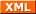Forum RSS feed ( https://gammon.com.au/rss/forum.xml )